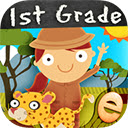Free editor online | DOC > | XLS > | PPT ># Animal Math First Grade Math Games Free in Chrome with OfAnimal Math First Grade Math Games Free Chrome web store extension

## DESCRIPTION

Run the Chrome online web store extension Animal Math First Grade Math Games Free using OffiDocs Chromium online.

Join math-explorer Emma in an all new adventure as she helps her animal friends complete over 100 first grade math games and find their way across the African wild! Playable by kids of all ages with lots of positive encouragement, professional narration and catchy music.

Cute characters include giraffes, meerkats, lions, hippos, zebras, warthogs, cheetahs and rhinos! Designed by parents and teachers, this app adheres to Common Core Standards for first grade math.

RELATES COUNTING TO MATH • Counting by 2s, 5s and 10s • Number sequences & patterns • Greater than, less than, equal to • Even & odd ALGEBRAIC THINKING • Find the missing sign • Understanding the equal sign • Determine the unknown number • Understanding place value ADDITION & SUBTRACTION • Number sentences • Objects, word problems, equations • True or false • Fluently add and subtract within 20 ADDITIONAL FEATURES: • Items, numbers and instructions are professionally narrated • Players are rewarded with positive encouragement • Parental controls: Turn off sounds, music and links to our other apps • We do not collect personal information from our users.

COMMON CORE STANDARDS • CCSS.

MATH.

CONTENT.

1.MD.

C.

4 - Represent and interpret data • CCSS.

MATH.

CONTENT.

1.G.

A.

1 - Reason with shapes and their attributes • CCSS.

MATH.

CONTENT.

1.OA.

C.

5 - Relate counting to addition and subtraction • CCSS.

MATH.

CONTENT.

1.NBT.

B.

2.A - Understanding place value in bundles of 10 • CCSS.

MATH.

CONTENT.

1.NBT.

B.

2.C - Understanding place value as multiples of 10 • CCSS.

MATH.

CONTENT.

1.OA.

D.

7 - Understand the equal sign, and if equations with addition and subtraction are true or false • CCSS.

MATH.

CONTENT.

1.NBT.

B.

3 - Comparing two-digit numbers • CCSS.

MATH.

CONTENT.

1.OA.

A.

1 - Use addition and subtraction within 20 to solve word problems • CCSS.

MATH.

CONTENT.

1.NBT.

C.

5 - Find 10 more or less than a two-digit number • CCSS.

MATH.

CONTENT.

1.NBT.

C.

6 - Subtract multiples of 10 from multiples of 10 • CCSS.

MATH.

CONTENT.

1.OA.

A.

2 - Solve word problems that call for addition of three whole numbers • CCSS.

MATH.

CONTENT.

1.OA.

D.

8 - Determine the unknown number in an addition or subtraction equation relating three whole numbers